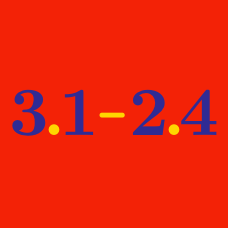Everyday Math

# Decimals: Level 4 Challenges

In the infinite series

$\frac { 1 }{ 9 } +\frac { 1 }{ 99 } +......+\cfrac { 1 }{ { 10 }^{ n }-1 } + \ldots$

at what place after the decimal point does the third 1 occur?

Note: The first 1 occurs at the first place after the decimal point.

Inspired by this problem

$0.\overline{0009182736455463728191}=\frac{1}{N}$

Given that $N$ is a 4-digit integer, find $N$.

Note: The repeated digits are from 00, 09, 18, ..., 81,(those multiple 9), followed by 91, the period is 22.

Consider the following series written in form of a decimal $A = \frac{1}{9} + \frac{1}{99} + \frac{1}{999} + .... + \frac{1}{10^{100} - 1}$

Find the digit in the $71$st place after the decimal point.

$0.\overline{00010203040506\ldots 969799}=\frac{1}{N}$

Given that $N$ is a 4-digit integer, find $N$.

Clarification: The repeated digits are from 00, 01, 02, ..., till 99, without 98, the period is 198.

What is the $1000^\text{th}$ digit to the right of the decimal point in the decimal representation of $(1+\sqrt{2})^{3000}$?

×